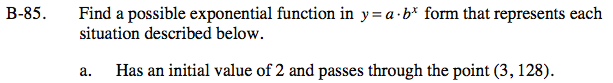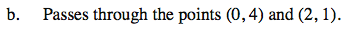### Home > A2C > Chapter 3 > Lesson 3.2.1 > Problem3-95

3-95.Since it has an initial value of 2, the value of a is 2.
Substitute this into the general equation: y = abx

Substitute the values 3 and 128 for x and y, respectively, then solve for b.Create two equations substituting the values of the ordered pairs for x and y into the equation y = abx.

Solve the system of equations for a and b.

Be sure you use your results for a and b to write the equation.